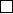1Some preliminary analysis

III Modular Forms and L-functions1.1 Characters of abelian groups
When we were young, we were forced to take some “applied” courses, and learnt
about these beasts known as Fourier transforms. At that time, the hope was
that we can leave them for the engineers, and never meet them ever again.
Unfortunately, it turns out Fourier transforms are also important in “pure”
mathematics, and we must understand them well.
Let’s recall how Fourier transforms worked. We had two separate but closely
related notions. First of all, we can take the Fourier transform of a function
f : R C. The idea is that we wanted to write any such function as
f(x) =
Z
−∞
e
2πiyx
ˆ
f(y) dy.
f
in the basis
χ
y
(
x
) =
e
2πiyx
.
We also could take the Fourier series of a periodic function, i.e. a function defined
on R/Z. In this case, we are trying to write our function as
f(x) =
X
n=−∞
c
n
e
2πinx
.
In this case, we are expanding our function in the basis
χ
n
(
x
) =
e
2πinx
. What
is special about these basis {χ
y
} and {χ
y
}?
We observe that
R
and
R/Z
are not just topological spaces, but in fact
abelian topological groups. These
χ
y
and
χ
n
are not just functions to
C
, but
continuous group homomorphisms to U(1)
C
. In fact, these give all continuous
group homomorphisms from R and R/Z to U(1).
Definition
(Character)
.
Let
G
be an abelian topological group. A (unitary)
character of
G
is a continuous homomorphism
χ
:
G
U(1), where U(1) =
{z
C | |z| = 1}.
To better understand Fourier transforms, we must understand characters,
and we shall spend some time doing so.
Example. For any group G, there is the trivial character χ
0
(g) 1.
Example. The product of two characters is a character.
Example. If χ is a character, then so is χ
, and χχ
= 1.
Thus, we see that the collection of all characters form a group under multi-
plication.
Definition
(Character group)
.
Let
G
be a group. The character group (or
Pontryagin dual )
ˆ
G is the group of all characters of G.
It is usually not hard to figure out what the character group is.
Example. Let G = R. For y R, we let χ
y
: R U(1) be
χ
y
(x) = e
2πixy
.
For each
y R
, this is a character, and all characters are of this form. So
ˆ
R
=
R
under this correspondence.
Example.
Take
G
=
Z
with the discrete topology. A character is uniquely
determined by the image of 1, and any element of U(1) can be the image of 1.
So we have
ˆ
G
=
U(1).
Example.
Take
G
=
Z/N Z
. Then the character is again determined by the
image of 1, and the allowed values are exactly the N th roots of unity. So
ˆ
G
=
µ
N
= {ζ C
×
: ζ
N
= 1}.
Example.
Let
G
=
G
1
× G
2
. Then
ˆ
G
=
ˆ
G
1
×
ˆ
G
2
. So, for example,
ˆ
R
n
=
R
n
.
Under this correspondence, a y R
n
corresponds to
χ
y
(x) = e
2πx·y
.
Example. Take G = R
×
. We have
G
=
1} ×R
×
>0
=
1} ×R,
where we have an isomorphism between
R
×
>0
=
R
by the exponential map. So
we have
ˆ
G
=
Z/2Z ×R.
Explicitly, given (ε, σ) Z/2Z ×R, then character is given by
x 7→ sgn(x)
ε
|x|
.
Note that
ˆ
G
has a natural topology for which the evaluation maps (
χ
ˆ
G
)
7→ χ
(
g
)
U(1) are all continuous for all
g
. Moreover, evaluation gives us a
map G
ˆ
ˆ
G.
Theorem
(Pontryagin duality)
.
Pontryagin duality If
G
is locally compact,
then G
ˆ
ˆ
G is an isomorphism.
Since this is a course on number theory, and not topological groups, we will
not prove this.
Proposition.
Let
G
be a finite abelian group. Then
|
ˆ
G|
=
|G|
, and
G
and
ˆ
G
are in fact isomorphic, but not canonically.
Proof.
By the classification of finite abelian groups, we know
G
is a product of
cyclic groups. So it suffices to prove the result for cyclic groups
Z/N Z
, and the
result is clear since
\
Z/N Z = µ
N
=
Z/N Z.